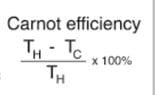# Violation of 2nd Law of Thermodynamics

• yinnxz
In summary,You can calculate efficiency for a Carnot engine only using the temperatures.However, the engine will be efficient even if the temperatures are negative.f

#### yinnxz

Homework Statement
Imagine that it were possible to construct
a reservoir at −5 K (below absolute zero).
Suppose you ran an engine and used the −5
K reservoir as the cold reservoir.
Would such an engine violate the second
law of thermodynamics?

1. Yes; the engine efficiency would be greater
than 100 %.
2. No, the engine efficiency would be high,
but reasonable.
Relevant Equations
η = Energy out/Total Energy * 100
I don't understand, can you calculate efficiency only using the temperature?

Homework Statement: Imagine that it were possible to construct
a reservoir at −5 K (below absolute zero).
Suppose you ran an engine and used the −5
K reservoir as the cold reservoir.
Would such an engine violate the second
law of thermodynamics?

1. Yes; the engine efficiency would be greater
than 100 %.
2. No, the engine efficiency would be high,
but reasonable.
Relevant Equations: η = Energy out/Total Energy * 100

I don't understand, can you calculate efficiency only using the temperature?
For a Carnot engine the efficiency does only depend on the temperatures.
Look it up in your book or on the internet.

PS: I can't make sense of the "relevant equation" you quote. The terminology is unclear. What is "total energy" and "energy out".

•russ_watters, yinnxz and topsquark
Hello @yinnxz ,##\qquad## !​

with exercises like this, it may be dificult to post your best effort (as required before the PF guidelines allow us to help !), but you can get started by at least posting the second law, so that we are all on the same page. And the 'relevant equation' is only complete if you clarify the variables.

##\ ##

•yinnxz
I don't understand, can you calculate efficiency only using the temperature?
You are right. It takes two temperatures. Two reservoirs. So pick a positive temperature, any positive temperature for the hot reservoir and see where that takes you.

There is an implicit assumption lurking here that heat will spontaneously flow from a positive temperature reservoir to a negative temperature reservoir. If you can Google well, you can discover definitions of thermodynamic temperature which make sense of negative temperatures. In the process, you may learn about the truth (or falsity) of that implicit assumption.

•yinnxz
This is a better equation I found. Sorry I should have taken a picture instead.

What I did was to plug in 100 on "TH" variable and the -5 K on the "TC" variable. I got an efficiency bigger than 100%. Would that make it violate the 2nd law that states that a system cant have 100% efficiency?

#### Attachments

•Screenshot_20230409_090353_Chrome.jpg
9.9 KB · Views: 21
Would that make it violate the 2nd law that states that a system cant have 100% efficiency?
In a previous post, I mentioned an implicit assumption that you are making: You are assuming that 100 degrees is hotter than -5 degrees.

"Hotter" in thermodynamics has a technical meaning -- heat will flow spontaneously from the "hotter" reservoir to the "colder" reservoir.

Negative temperatures (which are indeed possible) are hotter than all positive temperatures. The thermodynamic definition of temperature is weird and arguably backward. Reciprocal temperatures behave more sensibly in this regard.

•topsquark, BvU and yinnxz